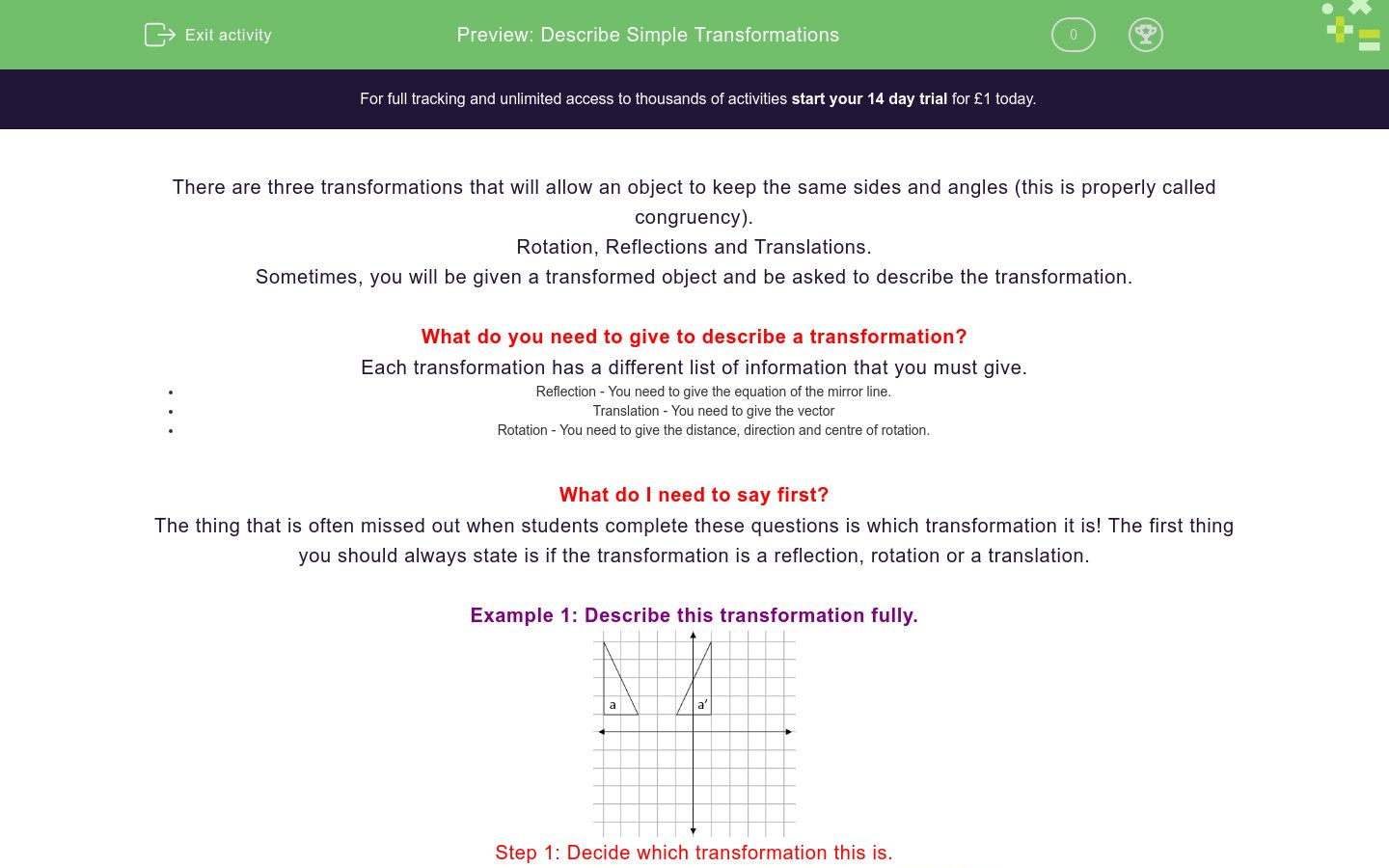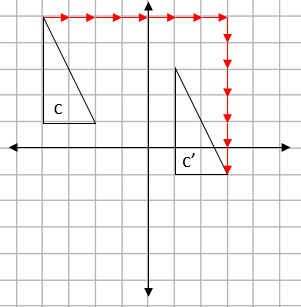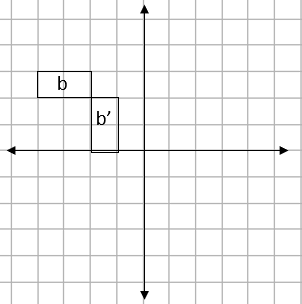# Describe Simple Transformations

In this worksheet, students practise describing simple transformations.Key stage:  KS 4

GCSE Subjects:   Maths

GCSE Boards:   AQA, Eduqas, Pearson Edexcel, OCR

Curriculum topic:   Geometry and Measures, Congruence and Similarity

Curriculum subtopic:   Properties and Constructions, Plane Isometric Transformations

Difficulty level:### QUESTION 1 of 10

There are three transformations that will allow an object to keep the same sides and angles (this is properly called congruency).

Rotation, Reflections and Translations.

Sometimes, you will be given a transformed object and be asked to describe the transformation.

What do you need to give to describe a transformation?

Each transformation has a different list of information that you must give.

• Reflection - You need to give the equation of the mirror line.
• Translation - You need to give the vector
• Rotation - You need to give the distance, direction and centre of rotation.

What do I need to say first?

The thing that is often missed out when students complete these questions is which transformation it is! The first thing you should always state is if the transformation is a reflection, rotation or a translation.

Example 1: Describe this transformation fully.Step 1: Decide which transformation this is.

a to a' is flipped. this means that the transformation is a reflection.

Step 2: Give the information we need.

For a reflection, we need a mirror line. This will always be exactly half way between the two shapes.This mirror line goes through the x axis at -2. This means the mirror line is x = -2

Example 2: Describe this transformation fully.Firstly, we can see that this is a rotation.The easiest way to find the centre of rotation is to trace the original shape and just try some points, when you find the one that works, that is the centre.

This transformation is described by...

Distance: 90°

Direction: Clockwise

Centre: (0,0)

Example 3: Describe this transformation fully.We can see that this is a translation.To describe a translation. We have to give a vector.

We can see from the arrows that are drawn that this is a movement of 7 to the right and 6 down.

This gives the vector...

 7 -6

Match the transformation with the word that describes it best.

## Column B

Rotation
Flip
Reflection
Turn
Translation
Slide

What type of transformation is shown here? Select all that applyReflection

Rotation

Translation

What is the equation of the mirror line for this reflection?What type of transformation is shown here? Select all that applyReflection

Rotation

Translation

Describe this rotation fully.Reflection

Rotation

Translation

What type of transformation is shown here? Select all that applyReflection

Rotation

Translation

Give the vector for this translation.a b

 Value a b

The three types of transformation that will give a congruent shape are...

 Value a b

What type of transformation is shown here? Select all that applyReflection

Rotation

Translation

Describe this rotation fully.Reflection

Rotation

Translation

• Question 1

Match the transformation with the word that describes it best.

## Column B

Rotation
Turn
Reflection
Flip
Translation
Slide
EDDIE SAYS
These words are really useful command words. You can easily spot if a shape has been turned, this means it has been rotated.
• Question 2

What type of transformation is shown here? Select all that applyReflection
Translation
EDDIE SAYS
This could either be a reflection as you could say the shape has flipped over, but you could also say that it has been slid downwards. It could also be a translation.
• Question 3

What is the equation of the mirror line for this reflection?y=2
EDDIE SAYS
The mirror line is always slap bang in the middle of the two shapes. For this question, it is a straight horizontal line that goes through the y - axis at 2
• Question 4

What type of transformation is shown here? Select all that applyRotation
EDDIE SAYS
This shape has been turned, this means it has to be a...
• Question 5

Describe this rotation fully.EDDIE SAYS
Remember that there are two different rotations we could state. It's either 90 CW or 270 ACW
• Question 6

What type of transformation is shown here? Select all that applyTranslation
EDDIE SAYS
This shape has been slid across and up, this means it is a...
• Question 7

Give the vector for this translation.a b

 Value a b
EDDIE SAYS
This shape has been moved 5 to the right and 3 up, this gives the vector...
• Question 8

The three types of transformation that will give a congruent shape are...

EDDIE SAYS
Congruent shapes just means that the angles and the sides of the transformed shapes stay the same. There is only one transformation that changes an object\'s size and that is enlargement. This means we must be looking for the other three.
• Question 9

What type of transformation is shown here? Select all that applyRotation
EDDIE SAYS
This shape has been turned, this means it has to be a...
• Question 10

Describe this rotation fully.EDDIE SAYS
Remember that there are two different rotations we could state. It's either 90 CW or 270 ACW
---- OR ----